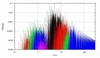## ATLAS OF TWO BODY MEAN MOTION RESONANCES IN THE SOLAR SYSTEM

Tabare Gallardo
Departamento Astronomia, Facultad de Ciencias, Uruguay
www.fisica.edu.uy/~gallardoBREAKING NEWS: There is an updated new website with new codes and plots HERE

The present plots, tables and programs give the location and strength of the MMRs between a massless particle and a planet. They were calculated considering fixed orbits for both two bodies. No variations in perihelion nor longitude of the node was considered. Mean semimajor axes were taken for the planets which were assumed with e=i=0. The strength SR(e,i,w) was calculated following Gallardo (2006, Atlas of MMRs in the solar system, Icarus 184, 29-38, preprint here). An improved and expanded version of the theory can be found in Gallardo 2019 (preprint arxiv here). It depends on the eccentricity, inclination and the argument of perihelion (w) of the body's orbit.

### Plots

(Mercury=red, Venus=green, Earth=blue, Mars=pink, Jupiter=black, Saturn=red, Uranus=green, Neptune=blue)

### How to ...

How to use all these numerology?
Suppose you are studying an asteroid or comet and you want to know if it is in a resonant motion. You have two possibilities:
• Look at the tables for the strongest resonances near the semimayor axis of the body's orbit. Then compute the corresponding critical angle
sigma = (p+q)*lambda_planet - p*lambda - q*longper
• Or run the program ATLAS (or SUPERATLAS) and compute the strength according to the (e,i,w) of the particle's orbit. This is the best choice. Then compute and look at the time evolution of the critical angles.

### Conventions

```
degree p<0: exterior resonances (|p+q|<|p|)
degree p>0: interior resonances (|p+q|>|p|)
order q>=0

Examples:

resonance 2:3 is an exterior resonance given by p=-3, q=1, then |-3+1|:|-3| = 2:3
resonance 3:2 is an interior resonance given by p=2, q=1, then |2+1|:|2| = 3:2
trojans 1:1 are given by p=-1, q=0
resonance 1:2N means Neptune makes 2 revolutions and the particle 1 revolution.
resonance 2:1N means Neptune makes 1 revolution and the particle 2 revolutions.

critical angle:    sigma = (p+q)*lambda_planet - p*lambda - q*longper
lambda = longper + mean anomaly

Minima of R(sigma) give the libration centers.

```

### Limitations

Planetary eccentricities were not taken into account so real motions should depart from this theory for very small eccentricities (e less than e_planet). Only one planet is taken into account then secular or resonant effects due to other planets can modify the theoretical libration centers given by the minima of R(sigma).